SSC BOARD PAPERS IMPORTANT TOPICS COVERED FOR BOARD EXAM 2024

### Polynomials Class 9th Mathematics Part I MHB Solution

##### Class 9th Mathematics Part I MHB Solution

###### Practice Set 3.1
Question 1.

State whether the given algebraic expressions are polynomials? Justify.

i. y + 1/y

ii. 2-5√x

iii. x2 + 7x + 9

iv. 2m-2 + 7m – 5

v. 10

(i) y + 1/y is not a polynomial because in this given equation the second term becomes 1 × y-1 where -1 is not a whole number. So the given algebraic expression is not a polynomial.

(ii) 2-5√x is not a polynomial because again the power of x is 1/2 which is not a whole number.

(iii) The given algebraic expression is a polynomial in one variable because all the powers of x are whole numbers.

(iv) This expression is not polynomial because the power of the first term is -2 which is not a whole number.

(v) The number 10 is a polynomial because 10 can be represented as follows: 10 × x0 which is equal to 10. So it is a polynomial.

Question 2.

Write the coefficient of in each of the given polynomial.

i. m3

ii.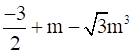iii.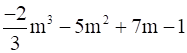i. The coefficient of any variable is the constant with which the variable is multiplied with. So the coefficient of m3 is one.

ii. The coefficient of m0 = -3/2

The coefficient of m = 1

The coefficient of m3 = -√3

iii. The coefficient of m0 = -1

The coefficient of m1 = 7

The coefficient of m2 = -5

The coefficient of m3 = -2/3

Question 3.

Write the polynomial in x using the given information.

i. Monomial with degree 7

ii. Binomial with degree 35

iii. Trinomial with degree 8

i. A polynomial is said to be monomial if it contains only one term in its entire expression. So the polynomial is as follows:

7x7

ii. A polynomial is said to be binomial if it contains only two term in its entire expression. So the polynomial is as follows:

2x35 + 7

iii. A polynomial is said to be trinomial if it contains only three term in its entire expression. So the polynomial is as follows:

2x8 + 7x + 3

Question 4.

Write the degree of the given polynomials.

i.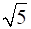ii. x0

iii. x2

iv.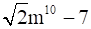v.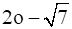vi. 7y – y3 + y5

vii. xyz + xy

viii. m3n7 – 3m5n + mn

i. The degree of a polynomial is the highest degree of its monomials (individual terms) with non-zero coefficients. This algebraic expression has zero degree.

ii. The degree of the given polynomial is zero.

iii. The degree of the given polynomial is two.

iv. The degree of the given polynomial is ten.

v. The degree of the given polynomial is one.

vi. The degree of the given polynomial is five.

vii. The degree of the given polynomial is three.

viii. The degree of the given polynomial is ten.

Question 5.

Classify the following polynomials as linear, quadratic and cubic polynomial.

i. 2x2 + 3x +1

ii. 5p

iii.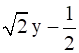iv.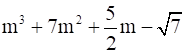v. a2

vi. 3r3

i. A polynomial is said to be quadratic if the highest power of the variable in the polynomial is two.

ii. The given polynomial is linear as the highest power of the variable is one.

iii. This polynomial is a linear one as the variable has the highest degree as one.

iv. This polynomial is a cubic polynomial because the highest power of the variable is three.

v. A polynomial is said to be quadratic if the highest power of the variable in the polynomial is two.

vi. This polynomial is a cubic polynomial because the highest power of the variable is three.

Question 6.

Write the following polynomials in standard form.

i. m3 + 3 + 5m

ii.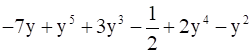i. In the standard form of any polynomial it is necessary for the power of the variable to go in descending order for each term.

So the standard form of this polynomial is as follows:

m3 + 5m + 3

ii. In the standard form of any polynomial it is necessary for the power of the variable to go in descending order for each term that is first term should consist of variable with highest power, second term should contain the variable with second highest power and so on.

So the standard form of this polynomial is as follows:

y5 + 2y4 + 3y3 –y2 -7y – 1/2

Question 7.

Write the following polynomials in coefficient form.

i. x3 – 2

ii. 5y

iii. 2m4 – 3m2 + 7

iv.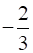i. In the coefficient form of the polynomial, the coefficient of each term of the variable present or absent is written inside the simple brackets. So the coefficient form is as follows:

⇒ x3 -2 = x3 + 0x2 + 0x – 2

∴ The given polynomial in coefficient form is:

(1, 0, 0, -2)

ii. In the coefficient form of the polynomial, the coefficient of each term of the variable present or absent is written inside the simple brackets. So the coefficient form is as follows:

⇒ 5y = 5.y + 0

Therefore, the given polynomial in coefficient form is:

(5, 0)

iii. In the coefficient form of the polynomial, the coefficient of each term of the variable present or absent is written inside the simple brackets. So, the coefficient form is as follows

2m4 – 3m2 + 7 = 2.m4 + 0.m3 – 3.m2 + 0.m + 7

Therefore, the given polynomial in coefficient form is:

(2, 0, -3, 0, 7)

iv. In the coefficient form of the polynomial, the coefficient of each term of the variable present or absent is written inside the simple brackets.

Now in this case all the powers of the variable are zero and only the constant is present.

So the coefficient form is as follows:

(-2/3)

Question 8.

Write the polynomials in index form.

i. (1, 2, 3)

ii. (5, 0, 0, 0, -1)

iii. (-2, 2, -2, 2)

i. The given representation is the coefficient form of the polynomial. So, the first coefficient denotes the highest power of the variable.

Writing in the index form, the polynomial is:

⇒ 1.x3 + 2x2 + 0.x + 3

⇒ x3 + 2x2 + 3

∴ Index form of polynomial is x3 + 2x2 + 3

ii. The given representation is the coefficient form of the polynomial. So the first coefficient denotes the highest power of the variable and the power of last term is always zero.

= 5.x4 +0.x3 + 0.x2 + 0.x – 1

= 5x4 – 1

Therefore, the index form of the polynomial is = 5x4 – 1

iii. The given representation is the coefficient form of the polynomial. So the first coefficient denotes the highest power of the variable and the power of last term is always zero.

= -2.x3 + 2.x2 – 2.x + 2

= -2x3 + 2x2 – 2x + 2

Therefore, the index form of the polynomial is = -2x3 + 2x2 – 2x + 2

Question 9.

Write the appropriate polynomials in the boxes.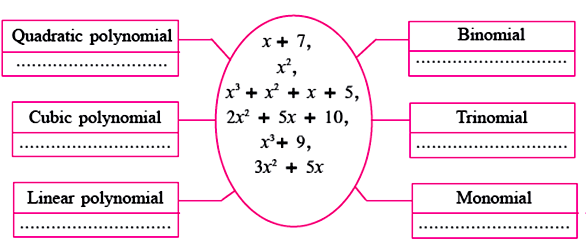A polynomial is said to be quadratic if the highest power of the variable in the polynomial is two.

The polynomial is said to be linear if the variable has the highest degree as one.

The polynomial is said to be cubic if the variable has the highest degree as three.

A polynomial is said to be monomial if it contains only one term in its entire expression.

A polynomial is said to be binomial if it contains only two terms in its entire expression.

A polynomial is said to be trinomial if it contains only three terms in its entire expression.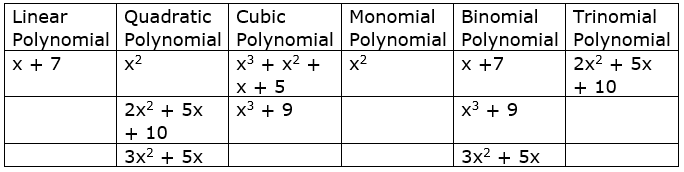###### Practice Set 3.2
Question 1.

Use the given letters to write the answer.

i. There are ‘a’ trees in the village Lat. If the number of trees increases every year by ‘b’, then how many trees will there be after ‘x’ years?

ii. For the parade there are y students in each row and x such row is formed. Then, how many students are there for the parade in all?

iii. The tens and units place of a two-digit number is m and n respectively. Write the polynomial which represents the two-digit number.

i. Given:

Total number of trees in the village = a

Increase in the number of trees by = b

To find: Number of trees after x years

Number of trees in x years = (a + b) x

ii. Given:

Number of students in each row = y

Number of rows of Students = x

So total number of students = x × y

= xy

iii. For a number in tens place should be multiplied with ten and for the number in units place should be multiplied by one. So the number that can be formed as follows:

Since m is in tens place and n is in units place,

10 × m + 1 × n

10m + n

So the polynomial representing the two digit number is = 10m + n

Question 2.

i. x3 – 2x2 – 9; 5x3 + 2x +9

ii.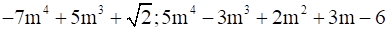iii. 2y2 + 5; 3y +9; 3y2 – 4y – 3

i.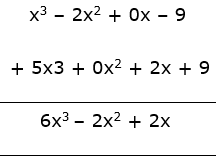The result = 6x3 – 2x2 + 2x

ii.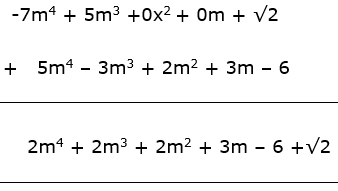The result = 2m4 + 2m3 + 2m2 + 3m – 6 +√2

iii.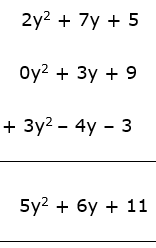The result = 5y2 + 6y + 11

Question 3.

Subtract the second polynomial from the first.

i.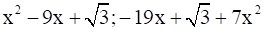ii. 2ab2 + 3a2b – 4ab; 3ab – 8ab2 +2a2b

In the subtraction process, the sign of the subtrahend that is the second polynomial is inverted and then the operation is carried out.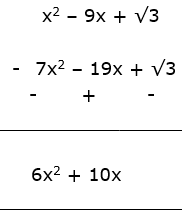The result = 6x2 + 10x

ii. In the subtraction process, the sign of the subtrahend that is the second polynomial is inverted and then the operation is carried out.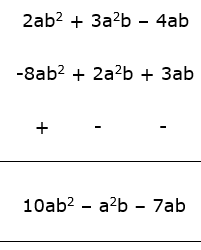The result = 10ab2 – a2b – 7ab

Question 4.

Multiply the given polynomials.

i. 2x; x2 – 2x

ii. x5 – 1; x3 + 2x3 + 2

iii. 2y + 1; y2 – 2y3 + 3y

The multiplication is as follows:

⇒ 2x × (x2 – 2x – 1)

⇒ 2x.x2 – 2x.2x – 2x.1

⇒ 2x2+1 – 4x1+1 – 2x

⇒ 2x3 – 4x2 – 2x

Therefore, the product = 2x3 – 4x2 – 2x

“.” represents multiplication

ii. The multiplication is as follows:

⇒ (x5 – 1) × (x3 + 2x3 + 2)

⇒ x5.x3 + 2x3.x5 + 2.x5 – 1.x3 – 1.2x3 – 1.2

⇒ x5+3 + 2x3 + 5 +2x5 – x3 – 2x3 – 2

⇒ x8 + 2x8 + 5 + 2x5 – x3 – 2x3 – 2

⇒ 3x8 + 2x5 + 3x3 + 3

The product is = 3x8 + 2x5 + 3x3 + 3

“.” represents multiplication

iii. The multiplication is as follows:

⇒ (2y + 1) × (y2 – 2y3 + 3y)

⇒ 2y.y2 – 2y3.2y + 3y.2y + 1.y2 – 1.2y3 + 1.3y

⇒ 2y2+1 – 4y3+1 + 6y1+1 + y2 – 2y3 + 3y

⇒ 2y3 – 4y4 + 6y2 + y2 – 2y3 + 3y

⇒ -4y4 + 7y2 + 3y

The product is = -4y4 + 7y2 + 3y

“.” represents multiplication

Question 5.

Divide first polynomial by second polynomial and write the answer in the form ‘Dividend = Divisor × Quotient + Remainder’.

i. x3 – 64; x – 4

ii. 5x5 + 4x4 – 3x3 + 2x2 + 2; x2 – x

i. The division is as follows: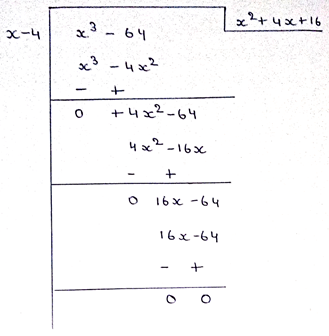x3-64 = [(x2 + 4x + 16) × (x-4)] + 0

x3-64 = (x2 + 4x + 16) × (x-4)

ii. The division is as follows: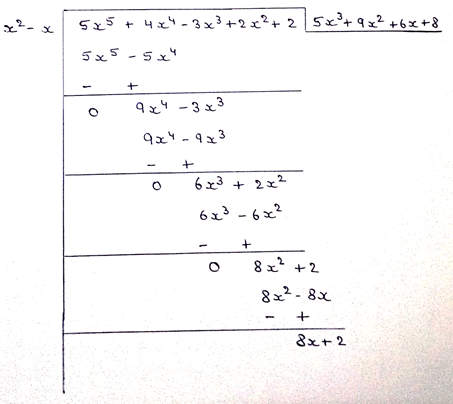5x5 + 4x4 – 3x3 + 2x2 + 2 = [(5x3 + 9x2 + 6x + 8) × (x2-x)] + 8x +2

Quotient = 5x3 + 9x2 + 6x + 8

Remainder = 8x + 2

Question 6.

Write down the information in the form of algebraic expression and simplify.

There is a rectangular farm with length (2a2 + 3b2) meter and breadth (a2 + b2) meter. The farmer used a square shaped plot of the farm to build a house. The side of the plot was (a2 - b2) meter. What is the area of the remaining part of the farm?

Given:

Length of the farm, l = (2a2 + 3b2) m

Breadth of the farm, b = (a2 + b2) m

Side of the square plot, s = (a2 - b2) m

To find: Area of remaining farm

Explain:

Area of Remaining Farm = Area of Rectangle – Area of Square

Area of Rectangle = l × b

= (2a2 + 3b2) × (a2 + b2)

= 2a2.a2 + 2a2.b2 + 3b2.a2 + 3b2.b2

= 2a4 + 5a2b2 + 3b4

Area of Square = s × s

= (a2 - b2) × (a2 - b2)

= a2.a2 – a2.b2 – a2.b2 + b2.b2

= a4 – 2a2b2 + b4

Area of Remaining Part = (2a4 + 5a2b2 + 3b4) – (a4 – 2a2b2 + b4)

= 2a4 + 5a2b2 + 3b4 - a4 + 2a2b2 - b4

= a4 + 7a2b2 + 2b4

Therefore, the area of remaining portion is = a4 + 7a2b2 + 2b4

###### Practice Set 3.3
Question 1.

Divide each of the following polynomials by synthetic division method and also by linear division method. Write the quotient and the remainder.

(2m2 – 3m + 10) ÷ (m – 5)

The linear division method is as follows: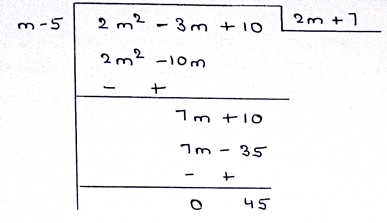Representing the polynomial in first polynomial in coefficient form:

⟹ 2.m2 – 3.m + 10

⟹ (2, – 3, 10)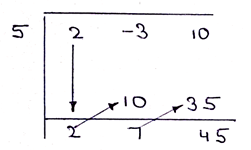Bring the first term as it is. Then multiply 5 with the value written in the bottom result area. Continue this until done.

Quotient = 2m + 7

Remainder = 45

Question 2.

Divide each of the following polynomials by synthetic division method and also by linear division method. Write the quotient and the remainder.

(x4 + 2x3 + 3x2 + 4x +5) ÷ (x + 2)

The linear division is as follows: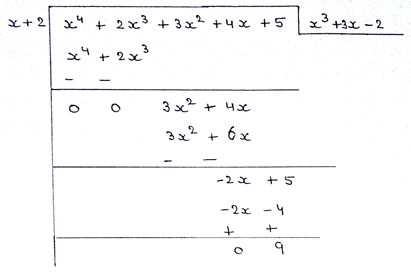Representing the polynomial in first polynomial in coefficient form:

⟹ 1.x4 + 2.x3 + 3x2 + 4x + 5

⟹ (1 2 3 4 5)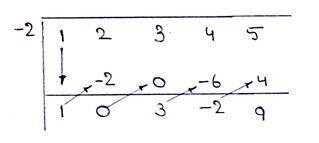So the final answer is written in the following form:

Quotient = x3 + 3x – 2

Remainder = 9

Question 3.

Divide each of the following polynomials by synthetic division method and also by linear division method. Write the quotient and the remainder.

(y3 – 216) ÷ (y – 6)

The linear division method is as follows: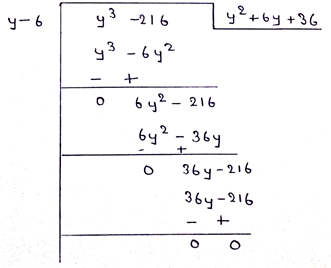Representing the polynomial in first polynomial in coefficient form:

⟹ 1.y3 + 0.y2 + 0.y – 216

⟹ (1, 0, 0, -216)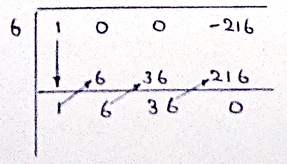So the final answer is written in the following form:

Quotient = y2 + 6y + 36

Remainder = 0

Question 4.

Divide each of the following polynomials by synthetic division method and also by linear division method. Write the quotient and the remainder.

(2x4 + 3x3 + 4x - 2x2) ÷ (x+3)